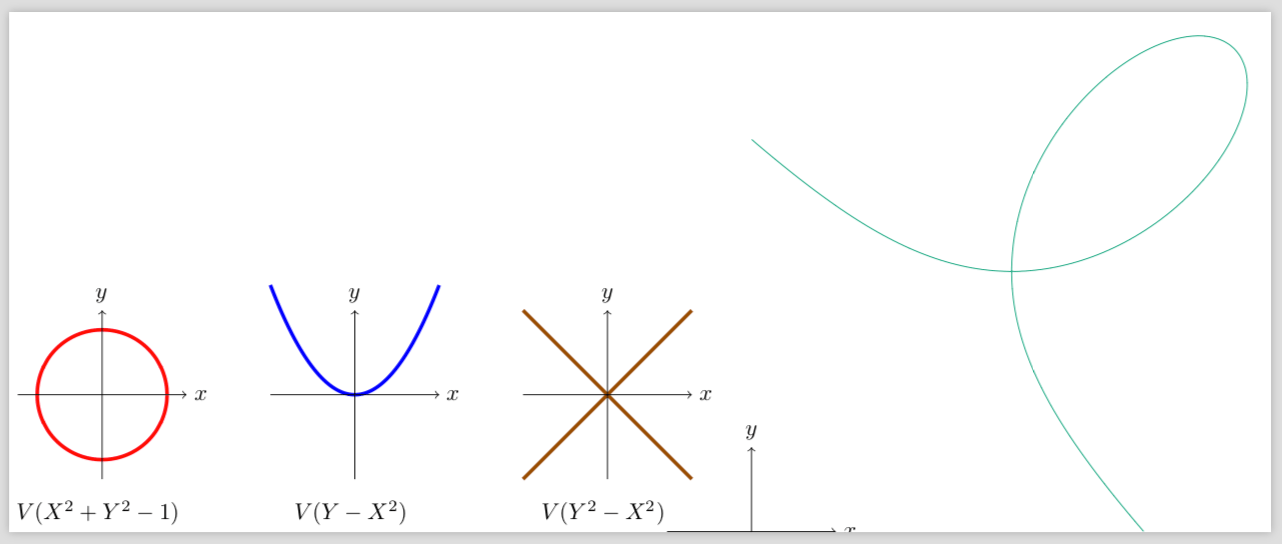# #StackBounty: #tikz-pgf #gnuplot #gnuplottex Same style with gnuplot than with normal graph with TikZ

### Bounty: 50

I’m learning how to use `gnuplot` with `TikZ`.

I would to draw next one to each other graphs of some algebraic curves. I’m starting with graphs that can be drawn without gnuplot (they are not implicit). I would like to draw next to them a implicit curve with the same style (same axis, centered and `ultra thick`).

Here is what I have achieved so far.

``````documentclass{standalone}

usepackage{tikz}
usepackage{gnuplot-lua-tikz}
usepackage[shell]{gnuplottex}
thispagestyle{empty}

begin{document}

begin{tikzpicture}
defsizeGraph{1.3}

draw[domain=-0.91:0.91, smooth, variable=x, red, ultra thick] plot ({x}, {sqrt(1-x*x)});
draw[domain=-1:-0.9, smooth, variable=x, red, ultra thick] plot ({x}, {sqrt(1-x*x)});
draw[domain=0.9:1, smooth, variable=x, red, ultra thick] plot ({x}, {sqrt(1-x*x)});

draw[domain=-0.91:0.91, smooth, variable=x, red, ultra thick] plot ({x}, {-sqrt(1-x*x)});
draw[domain=-1:-0.9, smooth, variable=x, red, ultra thick] plot ({x}, {-sqrt(1-x*x)});
draw[domain=0.9:1, smooth, variable=x, red, ultra thick] plot ({x}, {-sqrt(1-x*x)});

draw[->] (-sizeGraph,0) -- (sizeGraph,0) node[right] {\$x\$};
draw[->] (0,-sizeGraph) -- (0,sizeGraph) node[above] {\$y\$};

node [below=1.5cm, align=flush center]
{
\$V(X^2+Y^2-1)\$
};
end{tikzpicture}
begin{tikzpicture}
defsizeGraph{1.3}

draw[samples=1000, domain=-sizeGraph:sizeGraph, smooth, variable=x, blue, ultra thick] plot ({x}, {x*x});
draw[->] (-sizeGraph,0) -- (sizeGraph,0) node[right] {\$x\$};
draw[->] (0,-1.3) -- (0,1.3) node[above] {\$y\$};

node [below=1.5cm, align=flush center]
{
\$V(Y-X^2)\$
};
end{tikzpicture}
begin{tikzpicture}
defsizeGraph{1.3}

draw[samples=1000, domain=-sizeGraph:sizeGraph, smooth, variable=x, orange!60!black, ultra thick] plot ({x}, {x});
draw[samples=1000, domain=-sizeGraph:sizeGraph, smooth, variable=x, orange!60!black, ultra thick] plot ({x}, {-x});
draw[->] (-sizeGraph,0) -- (sizeGraph,0) node[right] {\$x\$};
draw[->] (0,-1.3) -- (0,1.3) node[above] {\$y\$};

node [below=1.5cm, align=flush center]
{
\$V(Y^2-X^2)\$
};
end{tikzpicture}
begin{tikzpicture}
defsizeGraph{1.3}

draw[->] (-sizeGraph,0) -- (sizeGraph,0) node[right] {\$x\$};
draw[->] (0,-1.3) -- (0,1.3) node[above] {\$y\$};

begin{gnuplot}[terminal=tikz,terminaloptions={size 8,8}]
set contour
set cntrparam levels incremental 0.0001, 0.0001, 0.0001
set view map
set view equal
unset surface
unset key
unset tics
unset border
set lmargin at screen 0
set rmargin at screen 1
set bmargin at screen 0
set tmargin at screen 1
set isosamples 1000,1000
set xrange [-3.5:3.5]
set yrange [-3.5:3.5]
set view 0,0
set cont base
splot x**3 + y**3 - 6*x*y
end{gnuplot}
end{tikzpicture}

end{document}
``````Can you help me?

Get this bounty!!!

This site uses Akismet to reduce spam. Learn how your comment data is processed.